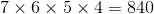# High School Math : Understanding Factorials

## Example Questions

### Example Question #1 : Other Factorials

What is the value of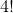?

24

4

12

6

10

24

Explanation:

! is the symbol for factorial, which means the product of the whole numbers less than the given number.

Thus,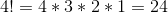### Example Question #1 : Understanding Factorials

What is the value of?Explanation:

By definition,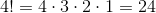### Example Question #3 : Multiplying And Dividing Factorials

Find the value of: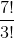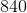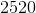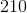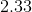Explanation:

The factorial sign (!) just tells us to multiply that number by every integer that leads up to it.  So,can also be written as: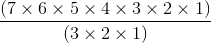To make this easier for ourselves, we can cancel out the numbers that appear on both the top and bottom: